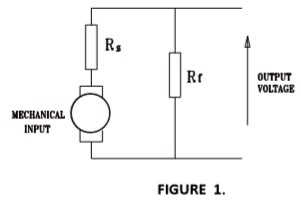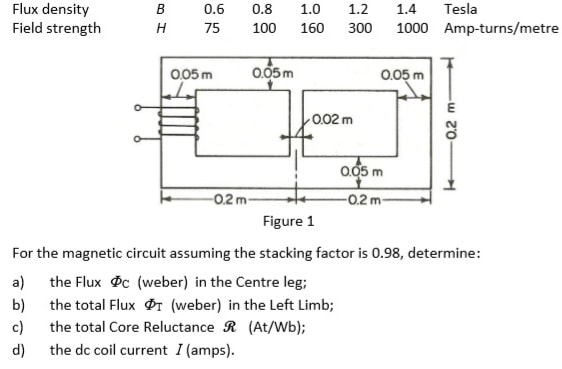# ELEN2001 Energy conversion assignment

```{`Electromagnetic & Electromechanical Energy Conversion [ELEN2001]
SEMESTER 2
Curtin University
School of Elec Eng, Comp and Math Sci (EECMS)
`}```

## Assignment

In all calculations the minimum amount of work (presentation) for each stage of the solution is:

• draw circuit
• state equation;
• transpose if necessary; (iv) substitute values into equation;

Only S.I. units are to be used for answers, eg. Newton-metres (Nm) for torque.

Assignment Office (204-Level 2 foyer).

Assignment must be submitted in a plastic sleeve, with a copy of the Assignment question sheets as obtained from blackboard and the university assignment attachment sheet.

Assignment may be hand written but must be using the requested formats.

NOTE: It is recognised that everyone has a different style and layout when completing homework questions and therefore COPYING and PLAGURISM will attract a marks penalty.

An example of the use of diagrams and solution layout for your assignment is shown below.

Note: Most problems can be solved by more than one way, any valid method is acceptable. It is unlikely that all students would use the same method or formatting. Identical method and formatting will be investigated as not being an individuals own work.

EXAMPLE

A 10 kW, 125 V, 4 pole, compound generator has rotational losses of PW = 580 W. The shunt field resistance Rf = 62.5 Ω, the lap connected armature resistance

Ra = 0.12 Ω, and the series field resistance Rs = 0.022 Ω.

Calculate the full load efficiency if the generator is connected long shunt.

Solution.

(i) CircuitTotal armature current:

(ii) Equation (iv) Substitute (v) Answer.QUESTION 1. [25 Marks]

In the magnetic circuit shown in Figure 1, a 400 turn coil on the left leg of the core is required to produce a flux of 1.4 mWb in the right leg. The thickness of the magnetic circuit is 0.04 m and is uniform throughout the core. The magnetic circuit is constructed with laminated silicon steel the characteristics of which are given below## QUESTION 2. [20 Marks]

A 40 kVA step up single phase transformer has the following name plate ratings:

 Power 40 kVA Frequency 50 Hz Phases 1 Primary voltage 415 V Secondary voltage 1100 V Insulation Class H

The results from an open circuit and short circuit tests are as follows:

Open circuit test

(Performed on secondary with primary open circuited)

VOC = 1100 V

IOC = 1.82 A

POC = 320 W

Short circuit test

(performed on primary with secondary short circuited)

VSC = 19.5 V

ISC = 96.4 A

PSC = 800 W

From these test results determine:

1. the approximate equivalent circuit parameters referred to the primary, and draw the circuit;
2. the efficiency at full load with a power factor of 8 leading;
3. the voltage regulation at full load with a power factor of 8 leading.

## QUESTION 3. [30 + 5 Marks]

(This problem illustrates the principles of dynamometer-type ac ammeter.) Two coils, one mounted on a stator and the other on a rotor, have self- and mutual inductances of

L11 = 3.5mH L22 = 1.8mH L12 = 2.1 cos θ mH

where θ is the angle between the axes of the coils. The coils are connected in series and carry a current

i = √2 I sin ωt

1. Derive an expression for the instantaneous torque T on the rotor as a function of the angular position θ;
2. Find an expression for the time-averaged torque Tavg as a function of θ;
3. Compute the numerical value of Tavg for I = 10 A and θ = 90°;
4. Sketch curves of Tavg versus θ for currents I = 5, 7.07, and 10 A;
5. A helical restraining spring which tends to hold the rotor at θ = 90° is now attached to the rotor. The restraining torque of the spring is proportional to the angular deflection from θ = 90° and is – 0.1 Nm when the rotor is turned to θ = 0°. Show on the curves of part (d) how you could find the angular position of the rotor-plus-spring combination for coil currents I = 5, 7.07, and 10 A. From your curves, estimate the rotor angle for each of these currents;
6. Write a MATLAB script to plot the angular position of the rotor as a function of rms current for 0 ≤ I ≤ 10 A [Bonus (+5)].

## QUESTION 4. [25 Marks]

A three phase induction motor with "Design B" characteristics has the motor name plate details are as follows:

 Power 7.5 kW Voltage 415 V Poles 4 Current 14.4 A Speed 1425 rpm Connection Delta Frequency 50 Hz NEMA Design B

The rotational losses (Friction and Windage losses) at full load are Pf/w = 0.315 kW.

 R1 = 1.745 Ω X1 = j7.90 Ω R2‘ = 2.37 Ω X2‘ = j11.85 Ω RC = 1670 Ω XM = j245 Ω

For the above motor:

1. Draw the complete equivalent circuit with all values indicated on the circuit including RC;

Using the above complete equivalent circuit and assuming rated voltage operation, determine:

1. the motor Starting Current;
2. the motor Starting Torque in Newton metres;
3. the motor Full Load Power Factor;
4. the motor Full Load percentage Efficiency;